# HTML5/JavaScript 图像自动Gamma校正 — 打造图像处理类库第一步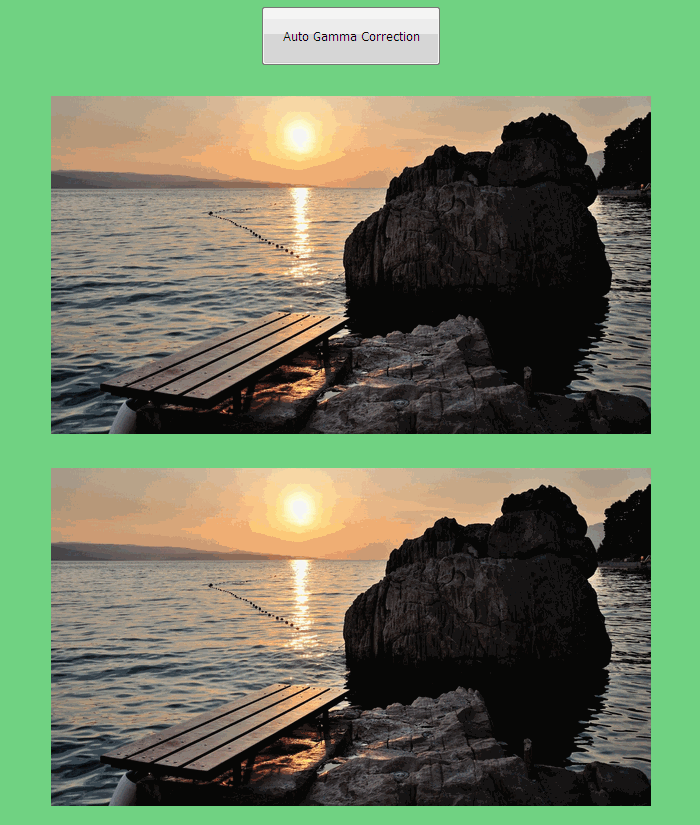1. 关于Gamma（伽马）
1.1 什么是Gamma

Gamma（伽马）是图像处理和视频制作中常用的技术用语。它定义了一个像素的数值和它的实际亮度之间的关系。从数学的角度来说，Gamma是用来改变某些输入值的“幂指数”。可以用如下简短的公式进行说明。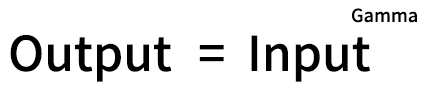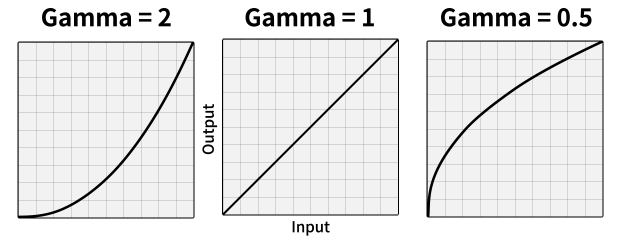①当Gamma值比1大时，在输入值相同的情况下，输出值减小；
②当Gamma值为1时，输出值不变；
③当Gamma值比1小时，在输入值相同的情况下，输出值增加。

1.2 什么是Gamma校正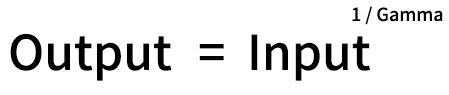1.3 为什么需要Gamma校正

① 人类对于外界刺激变化程度的感受，不是线性的，是指数形式的，如下图所示：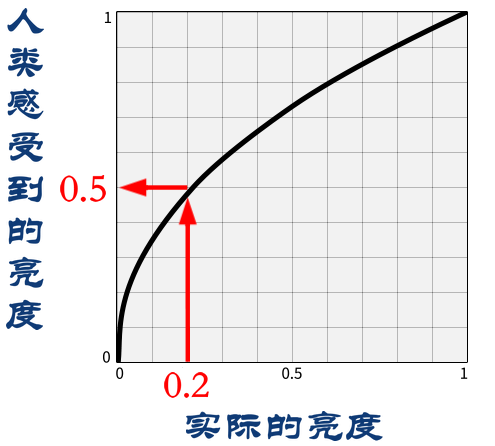② 图片文件的色阶很有限，24位色图片每个通道只有2^8个色阶，总共只能显示2^24种颜色；而且亮度级别过于庞大，如果按照线性方案进行存储，那么存储/传输的代价就会变得很昂贵。

2. 基本运用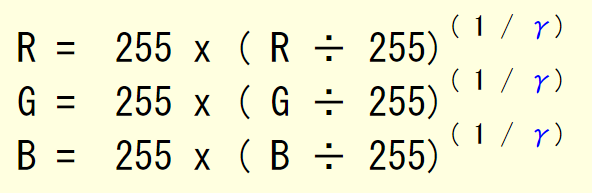3. 如何实现Gamma校正

function adjustImageGamma(img) {
image = img;
canvas = document.createElement('canvas');
canvas.id = img.id;
canvas.className = img.className;
//canvas.width = img.naturalWidth;
canvas.width = img.width;
//canvas.height = img.naturalHeight;
canvas.height = img.height;

ctx = canvas.getContext('2d');
//ctx.drawImage(img, 0, 0);
ctx.drawImage(img, 0, 0, img.width, img.height);

var image_parentNode = img.parentNode;
image_parentNode.replaceChild(canvas, img);

//imageData = ctx.getImageData(0, 0, img.naturalWidth, img.naturalHeight);
imageData = ctx.getImageData(0, 0, canvas.width, canvas.height);

var gammaVal = getGammaVal();
gammaCorrection = 1 / gammaVal;

setTimeout(function() {
//for ( y = 0; y < image.naturalHeight; y++) {
for ( y = 0; y < canvas.height; y++) {
//for ( x = 0; x < image.naturalWidth; x++) {
for ( x = 0; x < canvas.width; x++) {
var index = parseInt(x + canvas.width * y) * 4;
resetPixelColor(index);
}
}
ctx.putImageData(imageData, 0, 0);
//var dataURL = canvas.toDataURL('image/png');
}, 0);
}

function resetPixelColor(index) {
imageData.data[index + 0] = Math.pow(
(imageData.data[index + 0] / 255), gammaCorrection) * 255;
imageData.data[index + 1] = Math.pow(
(imageData.data[index + 1] / 255), gammaCorrection) * 255;
imageData.data[index + 2] = Math.pow(
(imageData.data[index + 2] / 255), gammaCorrection) * 255;
}

4. 如何实现自动Gamma校正

function getGammaVal() {
var pixData = imageData.data;
var grayNum = pixData.length / 4;
var totalGrayVal = 0;
for (var i = 0; i < pixData.length; i += 4) {
/* RGB to Luma:
* http://stackoverflow.com/questions/37159358/save-canvas-in-grayscale */
//var grayscale = pix[i] * 0.2126 + pix[i+1] * 0.7152 + pix[i+2] * 0.0722;
var grayscale = (pixData[i] + pixData[i+1] + pixData[i+2]) / 3;
totalGrayVal = totalGrayVal + grayscale;
}
var mean = totalGrayVal / grayNum;
var gammaVal = Math.log10(mean/255) / Math.log10(0.5);
return gammaVal;
}

https://github.com/mrdoob/three.js/wiki/How-to-run-things-locally我参考的这个链接里的内容，将程序运行于Server上，使图片和canvas处于同一个域，因为刚好安装了node.js的环境，所以我就直接 npm install http-server，然后再启动 http-server 加载html就可以了。

12-0304-22
01-07
11-156251
01-279
11-30847
04-162万+
12-09842
12-0823
06-052万+
12-02550
04-269922
10-21
03-01537
©️2020 CSDN 皮肤主题: 大白 设计师:CSDN官方博客